# Singapore MathThe American distributor for products from the country of Singapore, primarily math, but also a selection of science and English books. Since they are located only about five miles from us and they don't allow walk-in customers, we are their local retail outlet and have in-stock most of what they offer. Their site offers the Singapore scope and sequence, placement tests, and a variety of helpful information about their products.

Please Note: SingaporeMath.com asks us not to sell large quantities to public schools and school districts. We are happy to fill orders from private schools, individual teachers, and home school families, but if you are ordering for a school district, please contact Singapore directly through their website.

142 Items found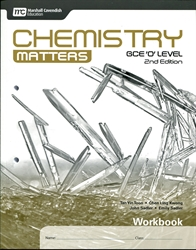Chemistry Matters - Workbook
from Singapore Math
in Old Edition Science (Location: ZCLE-SCI)
\$10.40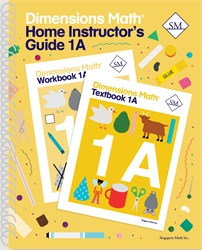Dimensions Math 1A - Home Instructor's Guide
from Singapore Math
in Dimensions Mathematics (Location: MAT-SMDM)
\$20.00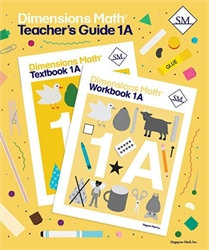Dimensions Math 1A - Teacher's Guide
from Singapore Math
in Dimensions Mathematics (Location: MAT-SMDM)
\$20.00 \$14.00 (1 in stock)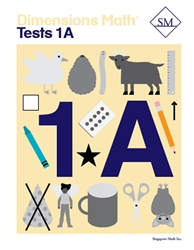Dimensions Math 1A - Tests
from Singapore Math
in Dimensions Mathematics (Location: MAT-SMDM)
\$12.00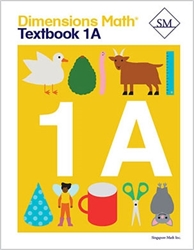Dimensions Math 1A - Textbook
from Singapore Math
in Dimensions Mathematics (Location: MAT-SMDM)
\$12.00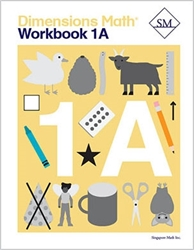Dimensions Math 1A - Workbook
from Singapore Math
in Dimensions Mathematics (Location: MAT-SMDM)
\$12.00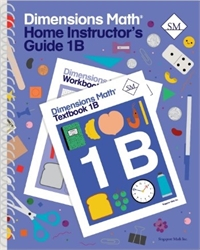Dimensions Math 1B - Home Instructor's Guide
from Singapore Math
in Dimensions Mathematics (Location: MAT-SMDM)
\$20.00Dimensions Math 1B - Teacher's Guide
from Singapore Math
in Dimensions Mathematics (Location: MAT-SMDM)
\$25.00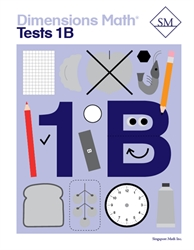Dimensions Math 1B - Tests
from Singapore Math
in Dimensions Mathematics (Location: MAT-SMDM)
\$12.00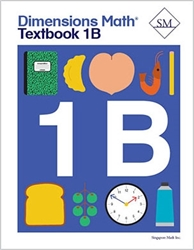Dimensions Math 1B - Textbook
from Singapore Math
in Dimensions Mathematics (Location: MAT-SMDM)
\$12.00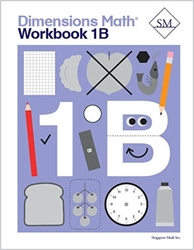Dimensions Math 1B - Workbook
from Singapore Math
in Dimensions Mathematics (Location: MAT-SMDM)
\$12.00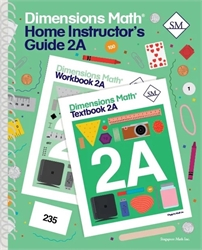Dimensions Math 2A - Home Instructor's Guide
from Singapore Math
in Dimensions Mathematics (Location: MAT-SMDM)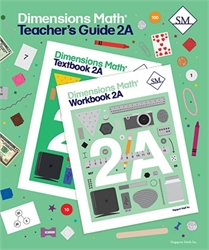Dimensions Math 2A - Teacher's Guide
from Singapore Math
in Dimensions Mathematics (Location: MAT-SMDM)
\$25.00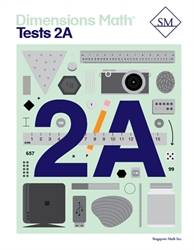Dimensions Math 2A - Tests
from Singapore Math
in Dimensions Mathematics (Location: MAT-SMDM)
\$12.00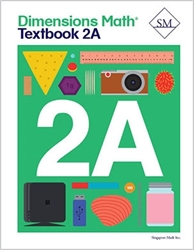Dimensions Math 2A - Textbook
from Singapore Math
in Dimensions Mathematics (Location: MAT-SMDM)
\$12.00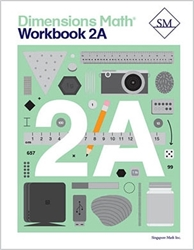Dimensions Math 2A - Workbook
from Singapore Math
in Dimensions Mathematics (Location: MAT-SMDM)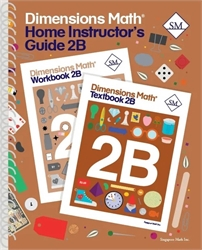Dimensions Math 2B - Home Instructor's Guide
from Singapore Math
in Dimensions Mathematics (Location: MAT-SMDM)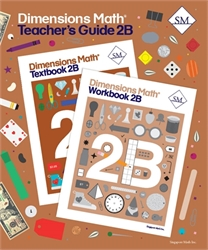Dimensions Math 2B - Teacher's Guide
from Singapore Math
in Dimensions Mathematics (Location: MAT-SMDM)
\$25.00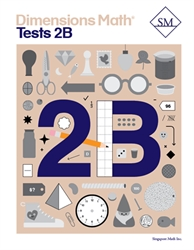Dimensions Math 2B - Tests
from Singapore Math
in Dimensions Mathematics (Location: MAT-SMDM)
\$12.00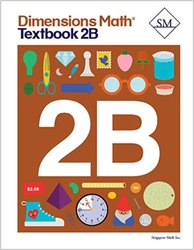Dimensions Math 2B - Textbook
from Singapore Math
in Dimensions Mathematics (Location: MAT-SMDM)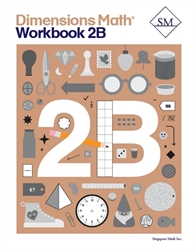Dimensions Math 2B - Workbook
from Singapore Math
in Dimensions Mathematics (Location: MAT-SMDM)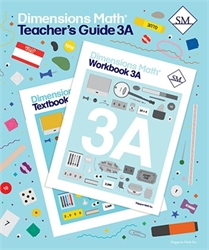Dimensions Math 3A - Teacher's Guide
from Singapore Math
in Dimensions Mathematics (Location: MAT-SMDM)
\$25.00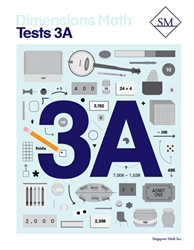Dimensions Math 3A - Tests
from Singapore Math
in Dimensions Mathematics (Location: MAT-SMDM)
\$12.00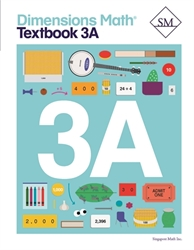Dimensions Math 3A - Textbook
from Singapore Math
in Dimensions Mathematics (Location: MAT-SMDM)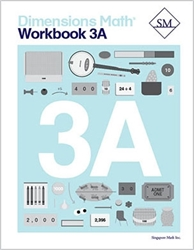Dimensions Math 3A - Workbook
from Singapore Math
in Dimensions Mathematics (Location: MAT-SMDM)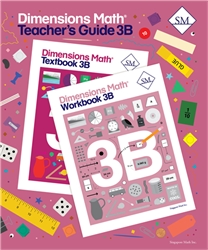Dimensions Math 3B - Teacher's Guide
from Singapore Math
in Dimensions Mathematics (Location: MAT-SMDM)
\$25.00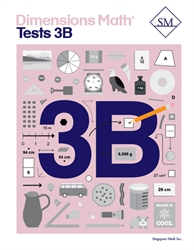Dimensions Math 3B - Tests
from Singapore Math
in Dimensions Mathematics (Location: MAT-SMDM)
\$12.00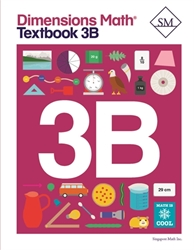Dimensions Math 3B - Textbook
from Singapore Math
in Dimensions Mathematics (Location: MAT-SMDM)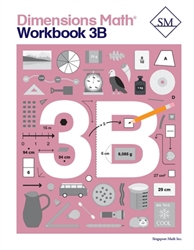Dimensions Math 3B - Workbook
from Singapore Math
in Dimensions Mathematics (Location: MAT-SMDM)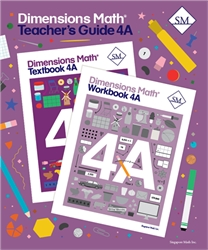Dimensions Math 4A - Teacher's Guide
from Singapore Math
in Dimensions Mathematics (Location: MAT-SMDM)
\$25.00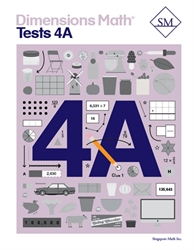Dimensions Math 4A - Tests
from Singapore Math
in Dimensions Mathematics (Location: MAT-SMDM)
\$12.00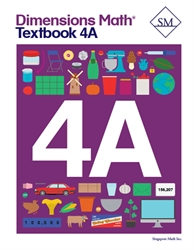Dimensions Math 4A - Textbook
from Singapore Math
in Dimensions Mathematics (Location: MAT-SMDM)
\$12.00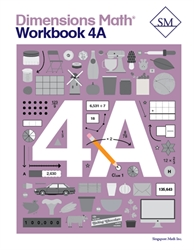Dimensions Math 4A - Workbook
from Singapore Math
in Dimensions Mathematics (Location: MAT-SMDM)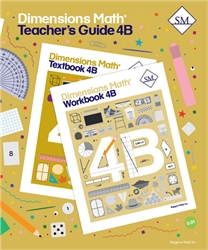Dimensions Math 4B - Teacher's Guide
from Singapore Math
in Dimensions Mathematics (Location: MAT-SMDM)
\$25.00 \$17.50 (1 in stock)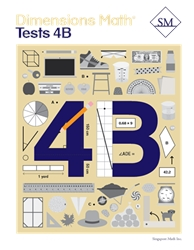Dimensions Math 4B - Tests
from Singapore Math
in Dimensions Mathematics (Location: MAT-SMDM)
\$12.00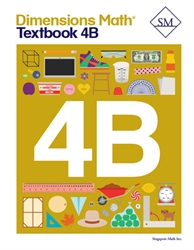Dimensions Math 4B - Textbook
from Singapore Math
in Dimensions Mathematics (Location: MAT-SMDM)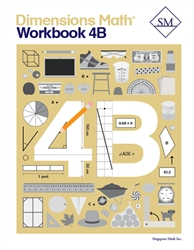Dimensions Math 4B - Workbook
from Singapore Math
in Dimensions Mathematics (Location: MAT-SMDM)
\$8.50 (1 in stock)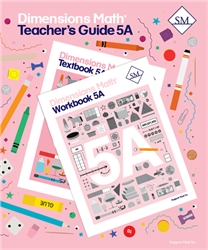Dimensions Math 5A - Teacher's Guide
from Singapore Math
in Dimensions Mathematics (Location: MAT-SMDM)
\$25.00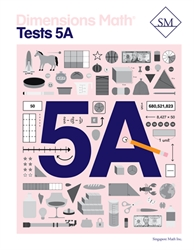Dimensions Math 5A - Tests
from Singapore Math
in Dimensions Mathematics (Location: MAT-SMDM)
\$12.00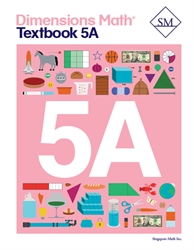Dimensions Math 5A - Textbook
from Singapore Math
in Dimensions Mathematics (Location: MAT-SMDM)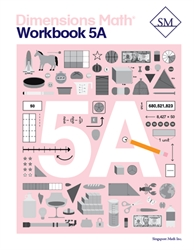Dimensions Math 5A - Workbook
from Singapore Math
in Dimensions Mathematics (Location: MAT-SMDM)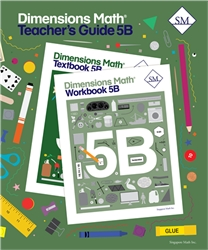Dimensions Math 5B - Teacher's Guide
from Singapore Math
in Dimensions Mathematics (Location: MAT-SMDM)
\$25.00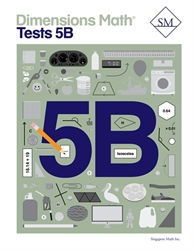Dimensions Math 5B - Tests
from Singapore Math
in Dimensions Mathematics (Location: MAT-SMDM)
\$12.00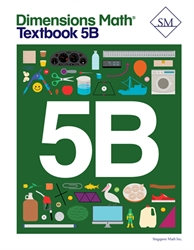Dimensions Math 5B - Textbook
from Singapore Math
in Dimensions Mathematics (Location: MAT-SMDM)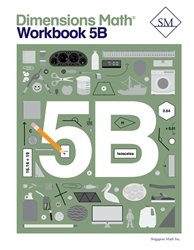Dimensions Math 5B - Workbook
from Singapore Math
in Dimensions Mathematics (Location: MAT-SMDM)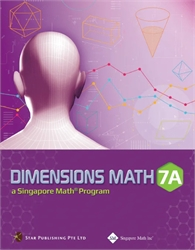Dimensions Math 7A - Textbook
from Singapore Math
in Dimensions Mathematics (Location: MAT-SMDM)
\$24.00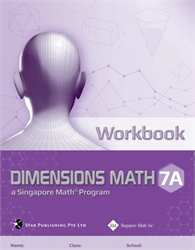Dimensions Math 7A - Workbook
from Singapore Math
in Dimensions Mathematics (Location: MAT-SMDM)
\$11.00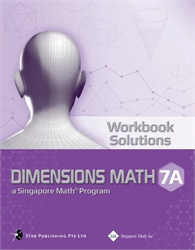Dimensions Math 7A - Workbook Solutions
from Singapore Math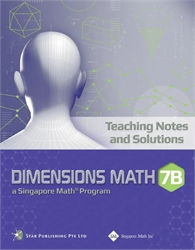Dimensions Math 7B - Teaching Notes & Solutions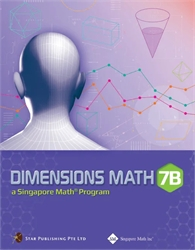Dimensions Math 7B - Textbook